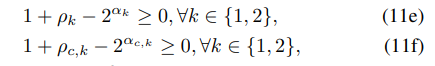# How to write 2 ^(optimzation variable)how can I write these constraints.

Note: that raw and alpha are variables need to be optimized.

You can enter this essentially as is, using `.^` in place of `^` to get vectorized version, as I have done…

``````C = 3; % made up value
cvx_begin
variables rho_k(2) alpha_k(2)
variables rho_c_k(C,2) alpha_c_k(C,2)
1 + rho_k - 2.^alpha_k >= 0
1 + rho_c_k - 2.^alpha_c_k >= 0
% the rest of your program
cvx_end
``````

Under the hood, CVX converts `2^x` to `exp(x*log(2))`, and uses the exponential cones to handle that. That is done automatically for you.

Thank you so much !!